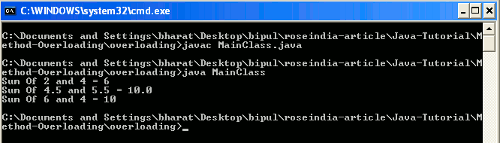Method overloading in Java is achieved due to the Java supports Polymorphism. Overloading of methods specifies the various methods defined with the same name. The concept of method overloading allows the Java programmer to use the method with the same name. But, these methods must be differentiated by their signature. Signature of method specifies the method's return type, number of arguments of method, data type of arguments of method. Constructors in Java is suitable example for understanding the concept of method overloading.

Overloading allows the Java programmer to accomplish the compile time polymorphism. Method overloading allows the user to use the various implementations of same name and give the desired output. This feature protects to know about the internal processing system from the external users.

Example

Here we are giving a simple example which will demonstrate you about the method overloading in Java. In this example we will create a Java class where we will use the concept of method overloading. As we have discussed above constructor of a class is also uses the concept of method overloading so, in this example we will create the various constructors of a class. Then we will create methods with the same name but, with the different return types and number of parameters.

```public class MethodOverloading {

int a;
int b;
double c;
double d;

{

}

{
this.a = a;
this.b = b;
}

{
int sum = a+b;
System.out.println("Sum Of "+a+" and "+b+" = "+sum);
}

public int add(int num1, int num2)
{
return num1+num2;
}
public double add(double num1, double num2)
{
return num1+num2;
}
}

```

MainClass.java

```public class MainClass {

public static void main(String args[])
{
int a = 6;
int b = 4;
double c = 4.5;
double d = 5.5;
System.out.println("Sum Of "+c+" and "+d+" = "+sum1);
System.out.println("Sum Of "+a+" and "+b+" = "+sum2);
}
}

```

Output

When you will execute the MainClass.java you will get the output as follows